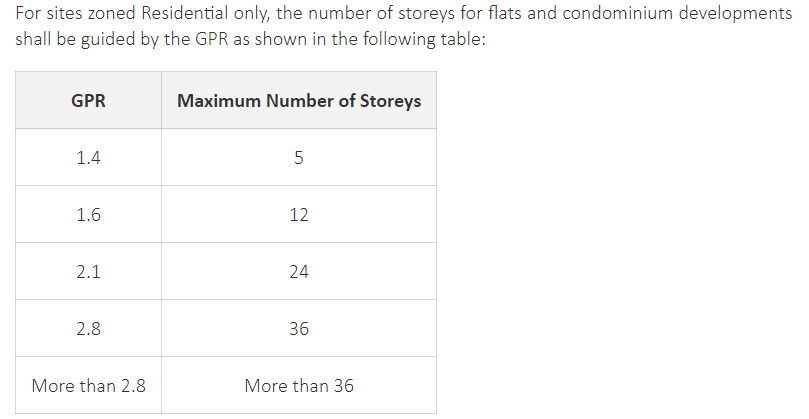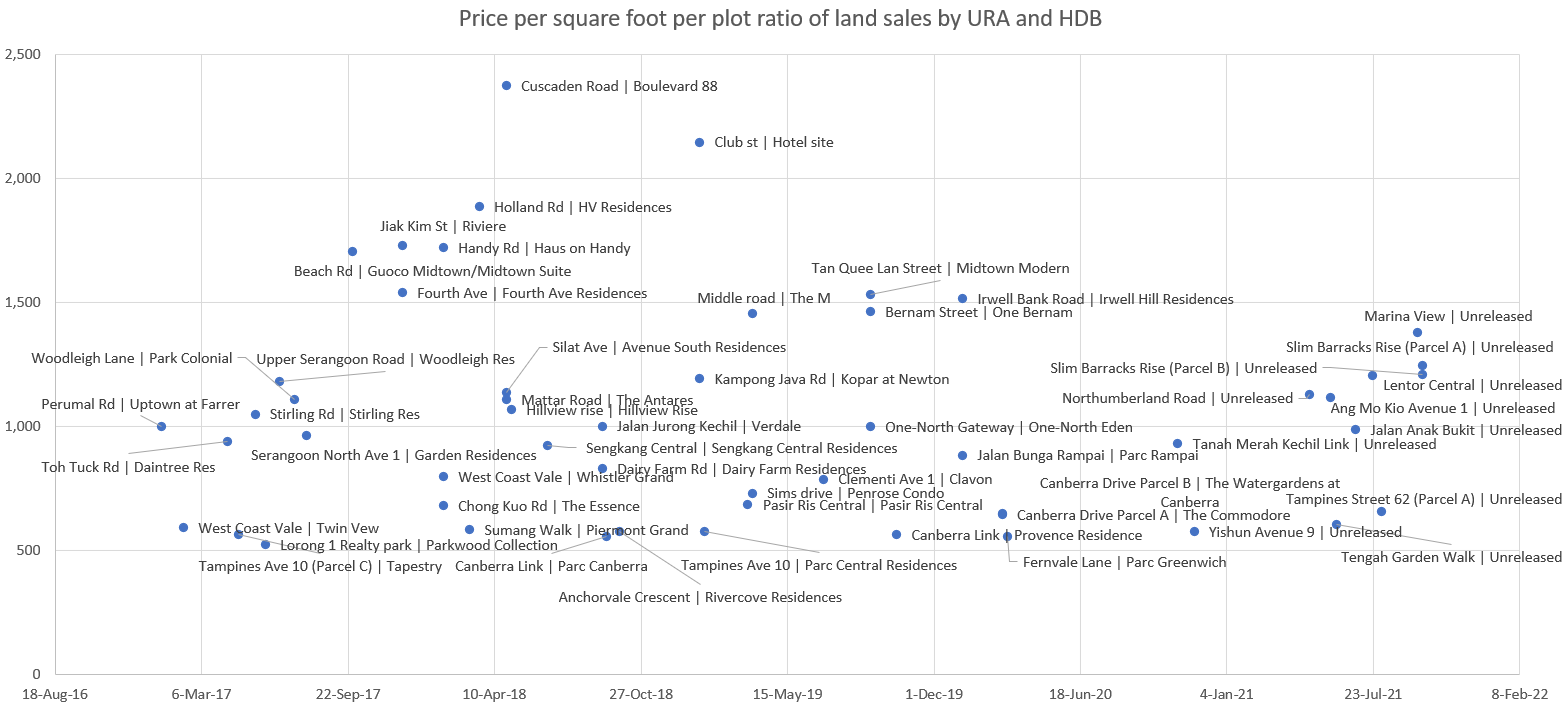Government land sales prices per square foot per plot ratio

Land sales by the government is a really important thing to track for those who are invested in the property market.

The following chart shows the price per square feet per plot ratio of land sales by URA and HDB from 2016.

For those confused by the term per square foot per plot ratio prices, an example here would suffice.

The URA designates a plot ratio for every piece of land.

The typical ratios are 1.4, 1.6, 2.1, 2.8 and more than 2.8.

A building can be built to total floor area of 14,000 sqft if the piece of land has a plot ratio of 1.4 and is 10,000 sqft in size. It can be built to 28,000 sqft if the plot ratio is 2.8 and the land size is 10,000 sqft.

The typical ratios areSo for example, a developer bids \$100 million for a piece of land. This piece of land is 100,000 sqft in size and has a plot ratio of 2.8. That means that the total floor area of the building on the piece of land can be a maximum of 280,000 sqft.

Based on the above, the developer would have paid a price per square foot per plot ratio of S\$100,000,000 divided by 100,000 sqft of land size = \$1,000 per square feet then divided by plot ratio of 2.8 = \$357 per square feet per plot ratio.

With this in mind, prices paid by developers are usually measured in per square feet per plot ratio terms to account for the fact that land of different sizes are sold, and each piece of land have different plot ratios.